Question

# The average score for games played in the NFL is 20.8 and the standard deviation is...

The average score for games played in the NFL is 20.8 and the standard deviation is 9.3 points. 46 games are randomly selected. Round all answers to 4 decimal places where possible and assume a normal distribution.

1. What is the distribution of ¯xx¯? ¯xx¯ ~ N( ___ , ____ )
2. What is the distribution of ∑x∑x? ∑x∑x ~ N( ____ , ____ )
3. P(¯xx¯ > 19.7432) = _____
4. Find the 62th percentile for the mean score for this sample size. _____
5. P(18.8432 < ¯xx¯ < 21.8856) = _____
6. Q3 for the ¯xx¯ distribution = _____
7. P(∑x∑x > 986.3872) = _____
8. For part c) and e), is the assumption of normal necessary? Yes or No _____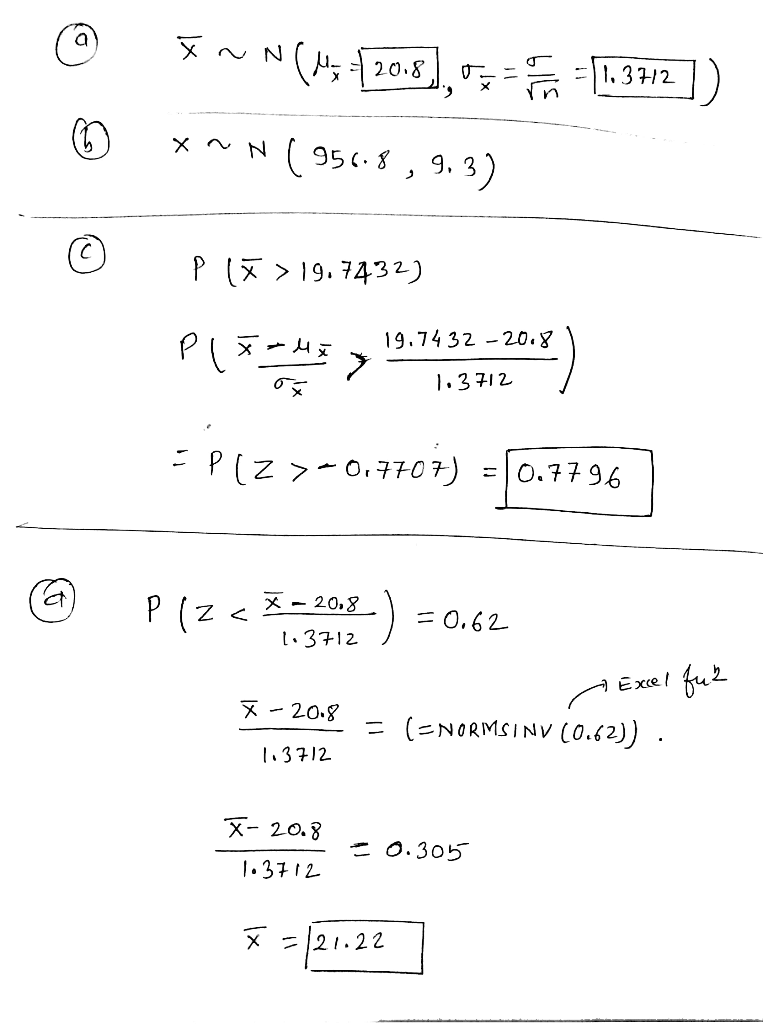#### Earn Coins

Coins can be redeemed for fabulous gifts.

Similar Homework Help Questions
• ### Q3(Sums) The average score for games played in the NFL is 21.7 and the standard deviation...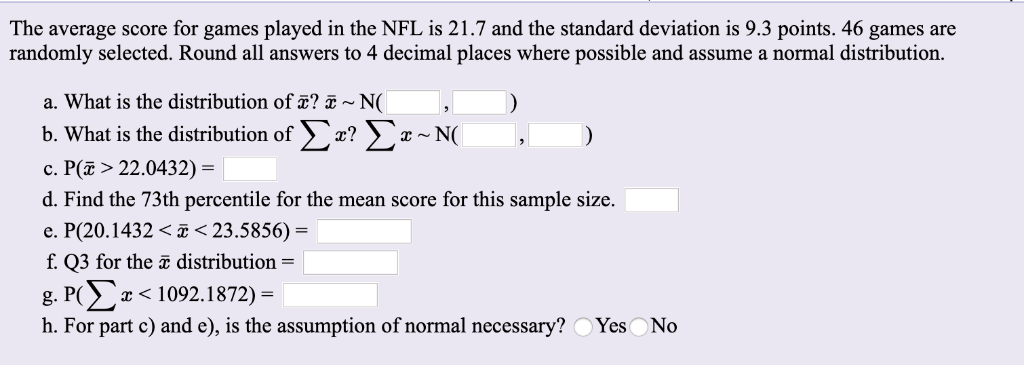Q3(Sums) The average score for games played in the NFL is 21.7 and the standard deviation is 9.3 points. 46 games are randomly selected. Round all answers to 4 decimal places where possible and assume a normal distribution. a. What is the distribution of a? 5 ~ N( Σ Σ--Ν( b.What is the distribution of c. P( > 22.0432) = d. Find the 73th percentile for the mean score for this sample size. e. P(20.1432 < E < 23.5856) =...

• ### The average score for games played in the NFL is 20.8 and the standard deviation is...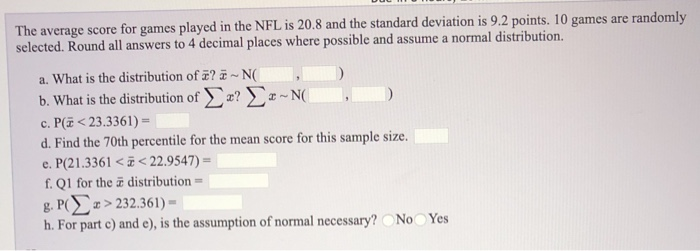The average score for games played in the NFL is 20.8 and the standard deviation is 9.2 points. 10 games are randomly selected. Round all answers to 4 decimal places where possible and assume a normal distribution. a. What is the distribution of ?-NO b. What is the distribution of x? -NO ) c. P(<23.3361) - d. Find the 70th percentile for the mean score for this sample size. e. P(21.3361 <i<22.9547) = f. Q1 for the distribution- g. PO...

• ### The average score for games played in the NFL is 22.1 and the standard deviation is...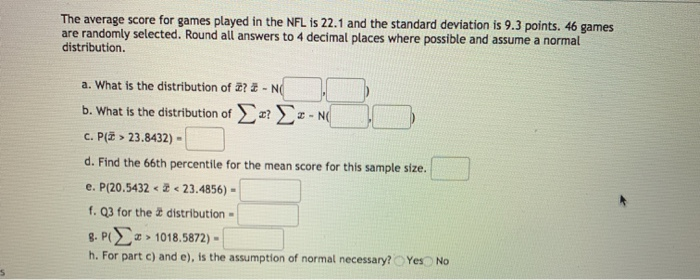The average score for games played in the NFL is 22.1 and the standard deviation is 9.3 points. 46 games are randomly selected. Round all answers to 4 decimal places where possible and assume a normal distribution. a. What is the distribution of 2?2- NO b. What is the distribution of r? -NG C. Plā > 23.8432) - d. Find the 66th percentile for the mean score for this sample size. e. P(20.5432 < 3 < 23.4856) - f. Q3...

• ### The average score for games played in the NFL is 21.9 and the standard deviation is 9.2 points. 44 games are randomly s...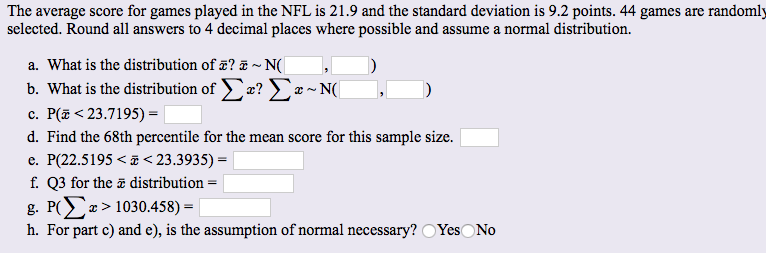The average score for games played in the NFL is 21.9 and the standard deviation is 9.2 points. 44 games are randomly selected. Round all answers to 4 decimal places where possible and assume a normal distribution. a. What is the distribution of ? ~ N(| b. What is the distribution of x? x~N(| c. P( <23.7195) d. Find the 68th percentile for the mean score for this sample size P(22.5195 23.3935) = e. f. Q3 for the distribution =...

• ### The average score for games played in the NFL is 21.8 and the standard deviation is...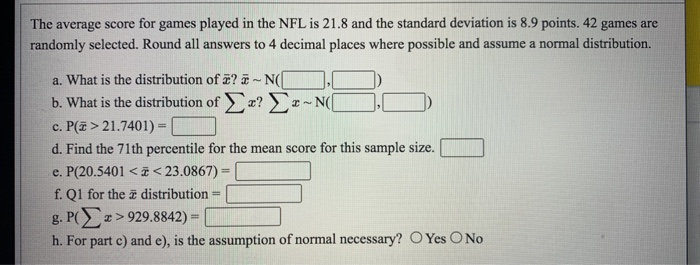The average score for games played in the NFL is 21.8 and the standard deviation is 8.9 points. 42 games are randomly selected. Round all answers to 4 decimal places where possible and assume a normal distribution. a. What is the distribution of a? -N([ b. What is the distribution of a? --N c. P> 21.7401) - d. Find the 71th percentile for the mean score for this sample size. e. P(20.5401 <ö<23.0867) = f. Q1 for the 2 distribution...

• ### help with e,f,g,h Td The average score for games played in the NFL is 21.8 and...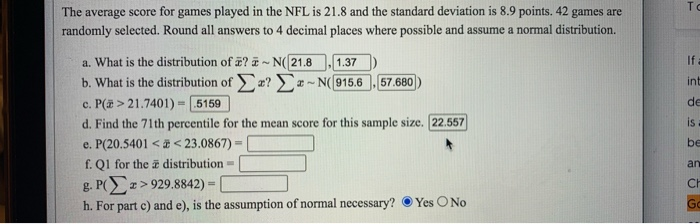help with e,f,g,h Td The average score for games played in the NFL is 21.8 and the standard deviation is 8.9 points. 42 games are randomly selected. Round all answers to 4 decimal places where possible and assume a normal distribution. If int de is a. What is the distribution of ? -N(21.8 1.37 b. What is the distribution of r? ~ ~ N(915.6 57.680 c. P(x > 21.7401) = .5159 d. Find the 71th percentile for the mean score...

• ### The average time to run the 5K fun run is 21 minutes and the standard deviation...

The average time to run the 5K fun run is 21 minutes and the standard deviation is 2.7 minutes. 17 runners are randomly selected to run the 5K fun run. Round all answers to 4 decimal places where possible and assume a normal distribution. What is the distribution of XX? XX ~ N(,) What is the distribution of ¯xx¯? ¯xx¯ ~ N(,) What is the distribution of ∑x∑x? ∑x∑x ~ N(,) If one randomly selected runner is timed, find the...

• ### The average price of a college math textbook is \$175 and the standard deviation is \$27....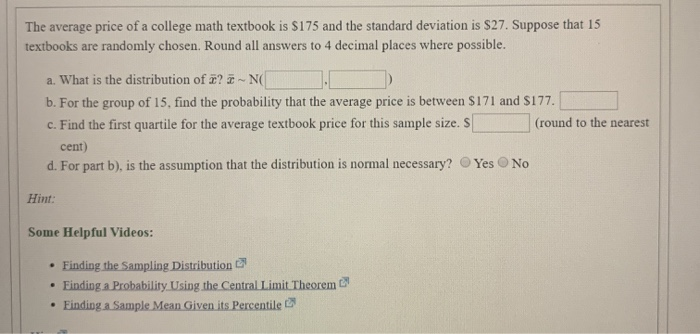The average price of a college math textbook is \$175 and the standard deviation is \$27. Suppose that 15 textbooks are randomly chosen. Round all answers to 4 decimal places where possible. a. What is the distribution of x? - NI b. For the group of 15, find the probability that the average price is between \$171 and \$177. c. Find the first quartile for the average textbook price for this sample size. S (round to the nearest cent) d....

• ### The average number of miles (in thousands) that a car's tire will function before needing replacement...

The average number of miles (in thousands) that a car's tire will function before needing replacement is 65 and the standard deviation is 16. Suppose that 40 randomly selected tires are tested. Round all answers to 4 decimal places where possible and assume a normal distribution. 1.) What is the distribution of XX? XX ~ N( , ) 2.) What is the distribution of ¯xx¯? ¯xx¯ ~ N( , ) 3.) If a randomly selected individual tire is tested, find...

• ### The average price of a college math textbook is \$162 and the standard deviation is \$24....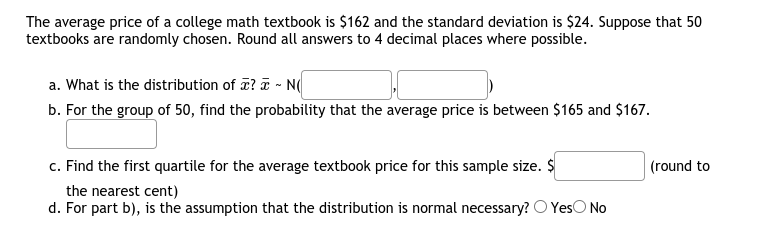The average price of a college math textbook is \$162 and the standard deviation is \$24. Suppose that 50 textbooks are randomly chosen. Round all answers to 4 decimal places where possible. a. What is the distribution of T?T-N b. For the group of 50, find the probability that the average price is between \$165 and \$167. (round to c. Find the first quartile for the average textbook price for this sample size. \$ the nearest cent) d. For part...